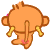﻿ 感觉这个问题挺可爱的 | Code Bye

# 感觉这个问题挺可爱的

8年前 (2016-04-25) 728次浏览

#include<stdio.h>
int main()
{
int a,i,b=0;
for(a=2;a<=1000;a++)
{
for(i=1;i<a;i++)
if(a%i==0)
b=b+i;
if(b==a)
{printf(“%d its factors are “,a);
for(i=1;i<a;i++)
if(a%i==0)
printf(“%d”,i);
printf(“\n”);}
}
return 0;
}

#include<stdio.h>
int main()
{
int a,i;
for(a=2;a<=1000;a++)
{ int b=0;
for(i=1;i<a;i++)
if(a%i==0)
b=b+i;
if(b==a)
{printf(“%d its factors are “,a);
for(i=1;i<a;i++)
if(a%i==0)
printf(“%d”,i);
printf(“\n”);}
}
return 0;
}

6 its factors are 123
28 its factors are 124714
496 its factors are 1248163162124248
Press any key to continue

10

10

10

10CodeBye 版权所有丨如未注明 , 均为原创丨本网站采用BY-NC-SA协议进行授权 , 转载请注明感觉这个问题挺可爱的

[1034331897@qq.com]